Plot frequencies of a variable as bar graph, histogram, box plot etc.

## Usage

plot_frq(
data,
...,
title = "",
weight.by = NULL,
title.wtd.suffix = NULL,
sort.frq = c("none", "asc", "desc"),
type = c("bar", "dot", "histogram", "line", "density", "boxplot", "violin"),
geom.size = NULL,
geom.colors = "#336699",
errorbar.color = "darkred",
axis.title = NULL,
axis.labels = NULL,
xlim = NULL,
ylim = NULL,
wrap.title = 50,
wrap.labels = 20,
grid.breaks = NULL,
expand.grid = FALSE,
show.values = TRUE,
show.n = TRUE,
show.prc = TRUE,
show.axis.values = TRUE,
show.ci = FALSE,
show.na = FALSE,
show.mean = FALSE,
show.mean.val = TRUE,
show.sd = TRUE,
drop.empty = TRUE,
mean.line.type = 2,
mean.line.size = 0.5,
inner.box.width = 0.15,
inner.box.dotsize = 3,
normal.curve = FALSE,
normal.curve.color = "red",
normal.curve.size = 0.8,
normal.curve.alpha = 0.4,
auto.group = NULL,
coord.flip = FALSE,
vjust = "bottom",
hjust = "center",
y.offset = NULL
)

## Arguments

data

A data frame, or a grouped data frame.

...

Optional, unquoted names of variables that should be selected for further processing. Required, if data is a data frame (and no vector) and only selected variables from data should be processed. You may also use functions like : or tidyselect's select_helpers.

title

Character vector, used as plot title. By default, response_labels is called to retrieve the label of the dependent variable, which will be used as title. Use title = "" to remove title.

weight.by

Vector of weights that will be applied to weight all cases. Must be a vector of same length as the input vector. Default is NULL, so no weights are used.

title.wtd.suffix

Suffix (as string) for the title, if weight.by is specified, e.g. title.wtd.suffix=" (weighted)". Default is NULL, so title will not have a suffix when cases are weighted.

sort.frq

Determines whether categories should be sorted according to their frequencies or not. Default is "none", so categories are not sorted by frequency. Use "asc" or "desc" for sorting categories ascending or descending order.

type

Specifies the plot type. May be abbreviated.

"bar"

for simple bars (default)

"dot"

for a dot plot

"histogram"

for a histogram (does not apply to grouped frequencies)

"line"

for a line-styled histogram with filled area

"density"

for a density plot (does not apply to grouped frequencies)

"boxplot"

for box plot

"violin"

for violin plots

geom.size

size resp. width of the geoms (bar width, line thickness or point size, depending on plot type and function). Note that bar and bin widths mostly need smaller values than dot sizes.

geom.colors

User defined color for geoms, e.g. geom.colors = "#0080ff".

errorbar.color

Color of confidence interval bars (error bars). Only applies to type = "bar". In case of dot plots, error bars will have same colors as dots (see geom.colors).

axis.title

Character vector of length one or two (depending on the plot function and type), used as title(s) for the x and y axis. If not specified, a default labelling is chosen. Note: Some plot types do not support this argument. In such cases, use the return value and add axis titles manually with labs, e.g.: plot.list[] + labs(x = ...) axis.labels character vector with labels used as axis labels. Optional argument, since in most cases, axis labels are set automatically. xlim Numeric vector of length two, defining lower and upper axis limits of the x scale. By default, this argument is set to NULL, i.e. the x-axis fits to the required range of the data. ylim numeric vector of length two, defining lower and upper axis limits of the y scale. By default, this argument is set to NULL, i.e. the y-axis fits to the required range of the data. wrap.title Numeric, determines how many chars of the plot title are displayed in one line and when a line break is inserted. wrap.labels numeric, determines how many chars of the value, variable or axis labels are displayed in one line and when a line break is inserted. grid.breaks numeric; sets the distance between breaks for the axis, i.e. at every grid.breaks'th position a major grid is being printed. expand.grid logical, if TRUE, the plot grid is expanded, i.e. there is a small margin between axes and plotting region. Default is FALSE. show.values Logical, whether values should be plotted or not. show.n logical, if TRUE, adds total number of cases for each group or category to the labels. show.prc logical, if TRUE (default), percentage values are plotted to each bar If FALSE, percentage values are removed. show.axis.values logical, whether category, count or percentage values for the axis should be printed or not. show.ci Logical, if TRUE), adds notches to the box plot, which are used to compare groups; if the notches of two boxes do not overlap, medians are considered to be significantly different. show.na logical, if TRUE, NA's (missing values) are added to the output. show.mean Logical, if TRUE, a vertical line in histograms is drawn to indicate the mean value of the variables. Only applies to histogram-charts. show.mean.val Logical, if TRUE (default), the mean value is printed to the vertical line that indicates the variable's mean. Only applies to histogram-charts. show.sd Logical, if TRUE, the standard deviation is annotated as shaded rectangle around the mean intercept line. Only applies to histogram-charts. drop.empty Logical, if TRUE and the variable's values are labeled, values / factor levels with no occurrence in the data are omitted from the output. If FALSE, labeled values that have no observations are still printed in the table (with frequency 0). mean.line.type Numeric value, indicating the linetype of the mean intercept line. Only applies to histogram-charts and when show.mean = TRUE. mean.line.size Numeric, size of the mean intercept line. Only applies to histogram-charts and when show.mean = TRUE. inner.box.width width of the inner box plot that is plotted inside of violin plots. Only applies if type = "violin". Default value is 0.15 inner.box.dotsize size of mean dot insie a violin or box plot. Applies only when type = "violin" or "boxplot". normal.curve Logical, if TRUE, a normal curve, which is adjusted to the data, is plotted over the histogram or density plot. Default is FALSE. Only applies when histograms or density plots are plotted (see type). normal.curve.color Color of the normal curve line. Only applies if normal.curve = TRUE. normal.curve.size Numeric, size of the normal curve line. Only applies if normal.curve = TRUE. normal.curve.alpha Transparancy level (alpha value) of the normal curve. Only applies if normal.curve = TRUE. auto.group numeric value, indicating the minimum amount of unique values in the count variable, at which automatic grouping into smaller units is done (see group_var). Default value for auto.group is NULL, i.e. auto-grouping is off. See group_var for examples on grouping. coord.flip logical, if TRUE, the x and y axis are swapped. vjust character vector, indicating the vertical position of value labels. Allowed are same values as for vjust aesthetics from ggplot2: "left", "center", "right", "bottom", "middle", "top" and new options like "inward" and "outward", which align text towards and away from the center of the plot respectively. hjust character vector, indicating the horizontal position of value labels. Allowed are same values as for vjust aesthetics from ggplot2: "left", "center", "right", "bottom", "middle", "top" and new options like "inward" and "outward", which align text towards and away from the center of the plot respectively. y.offset numeric, offset for text labels when their alignment is adjusted to the top/bottom of the geom (see hjust and vjust). ## Value A ggplot-object. ## Note This function only works with variables with integer values (or numeric factor levels), i.e. scales / centered variables with fractional part may result in unexpected behaviour. ## Examples library(sjlabelled) data(efc) data(iris) # simple plots, two different notations plot_frq(iris, Species)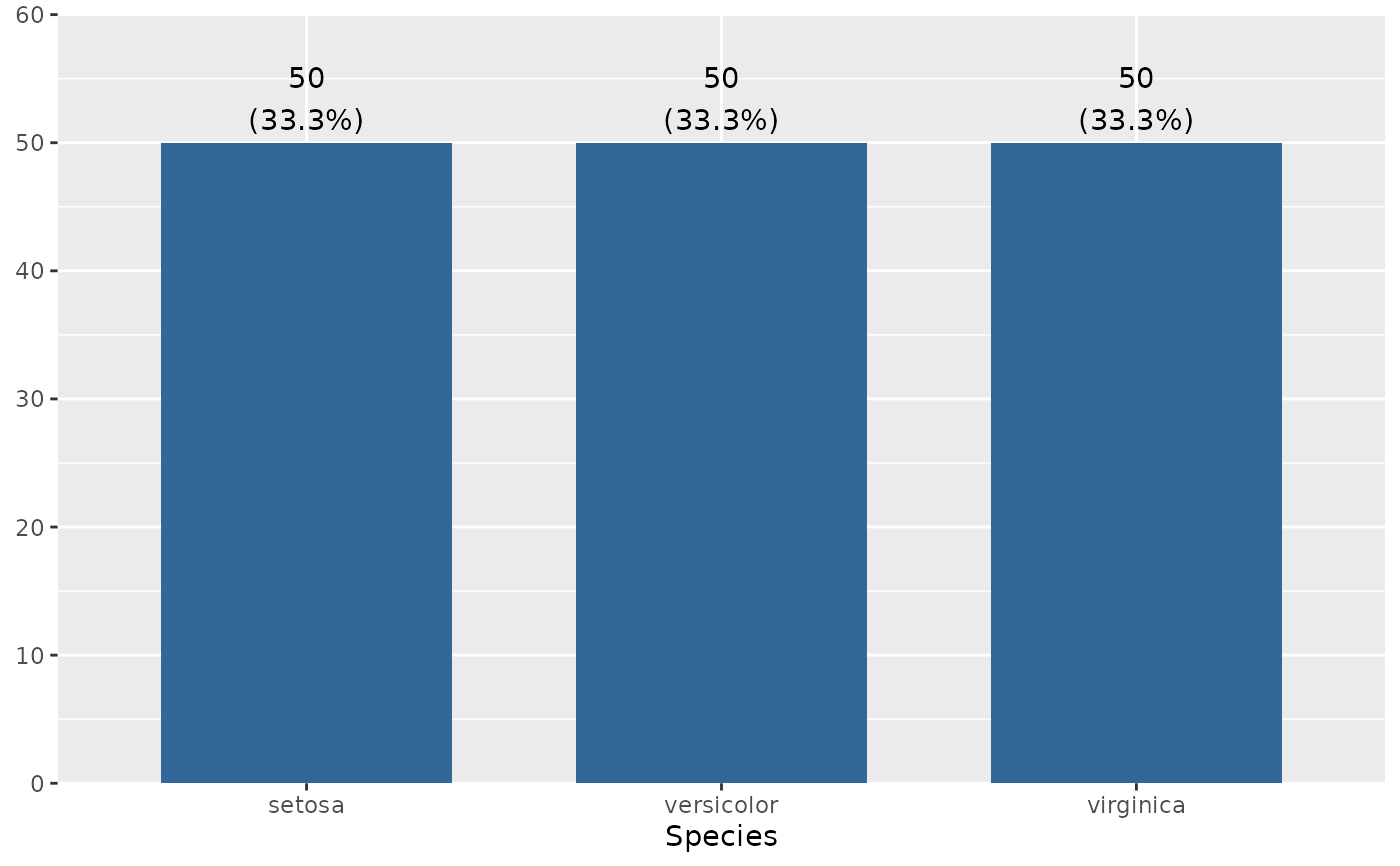plot_frq(efctot_sc_e)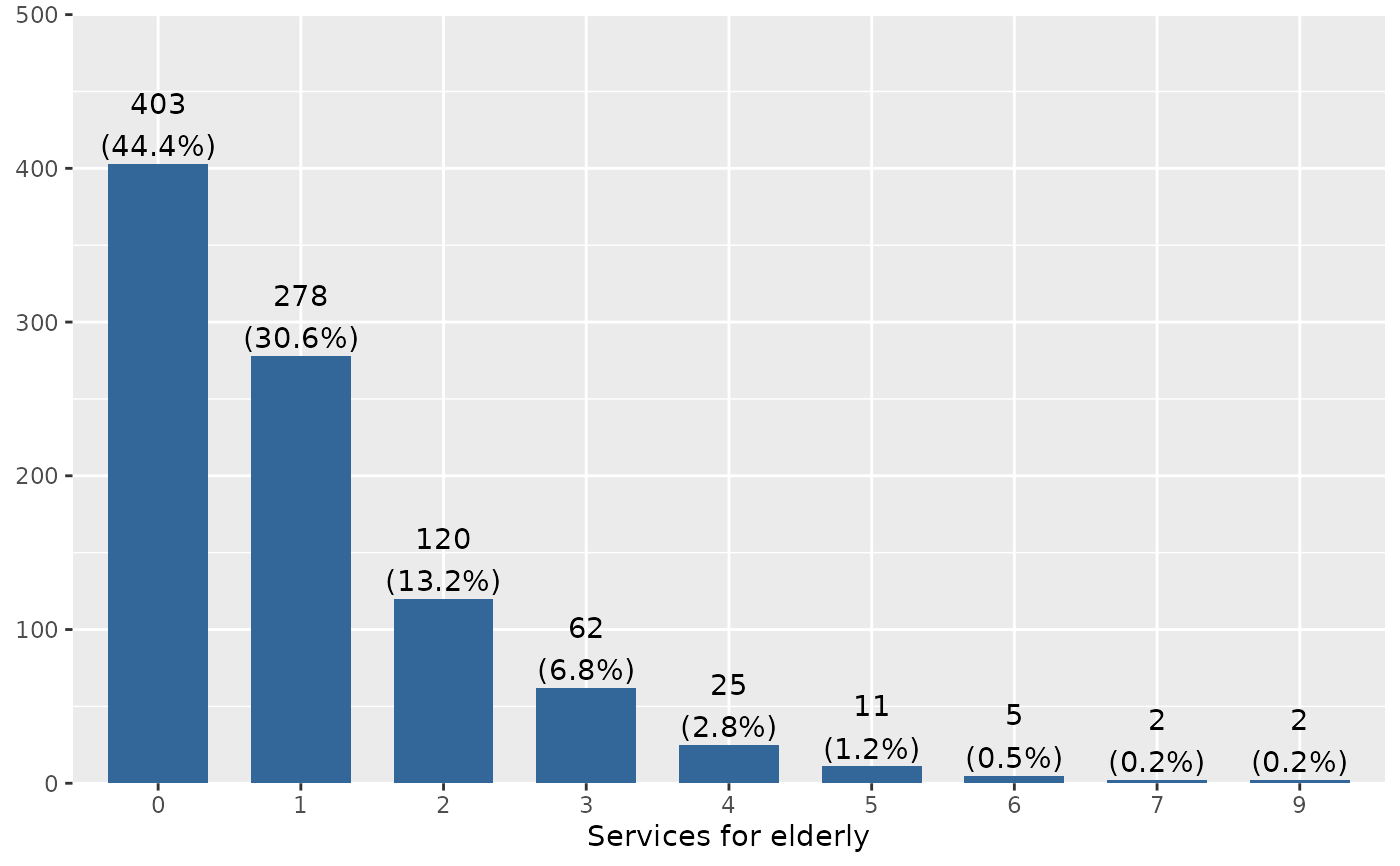# boxplot
plot_frq(efc$e17age, type = "box") #> Warning: The fun.y argument of stat_summary() is deprecated as of ggplot2 3.3.0. #> ℹ Please use the fun argument instead. #> ℹ The deprecated feature was likely used in the sjPlot package. #> Please report the issue at <https://github.com/strengejacke/sjPlot/issues>.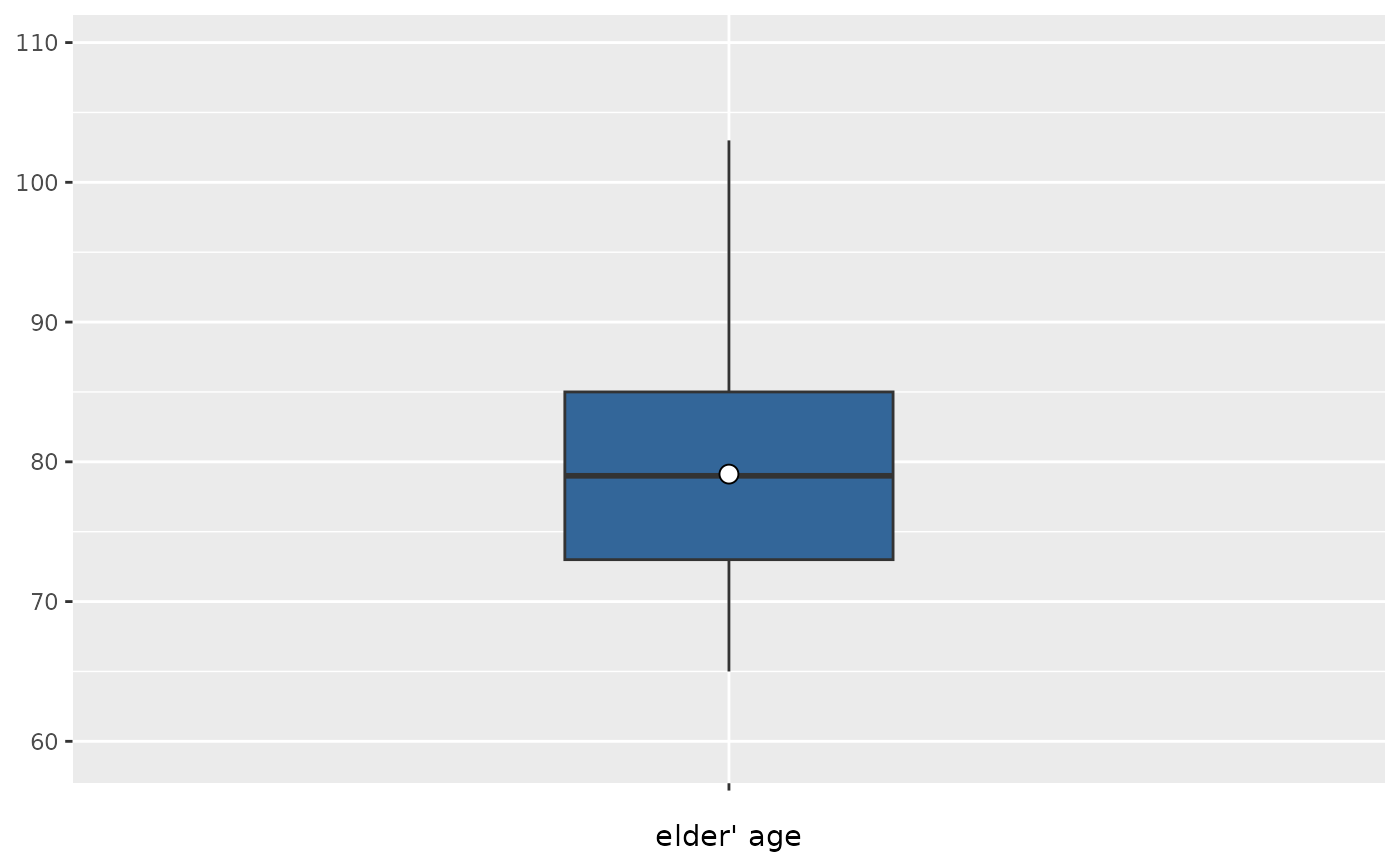if (require("dplyr")) { # histogram, pipe-workflow efc %>% dplyr::select(e17age, c160age) %>% plot_frq(type = "hist", show.mean = TRUE) # bar plot(s) plot_frq(efc, e42dep, c172code) } #> Loading required package: dplyr #> #> Attaching package: ‘dplyr’ #> The following object is masked from ‘package:sjlabelled’: #> #> as_label #> The following objects are masked from ‘package:stats’: #> #> filter, lag #> The following objects are masked from ‘package:base’: #> #> intersect, setdiff, setequal, union #> []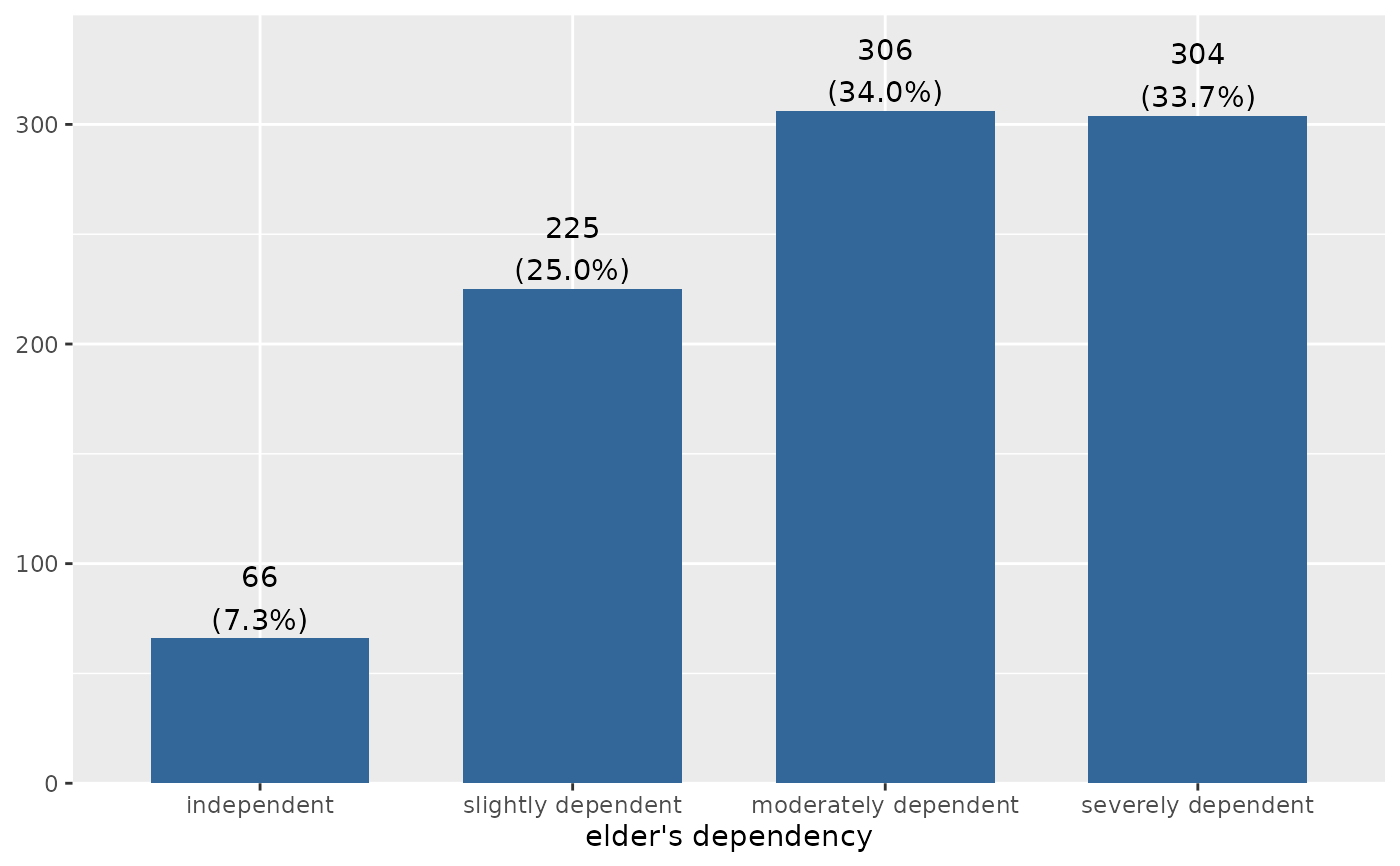#> #> []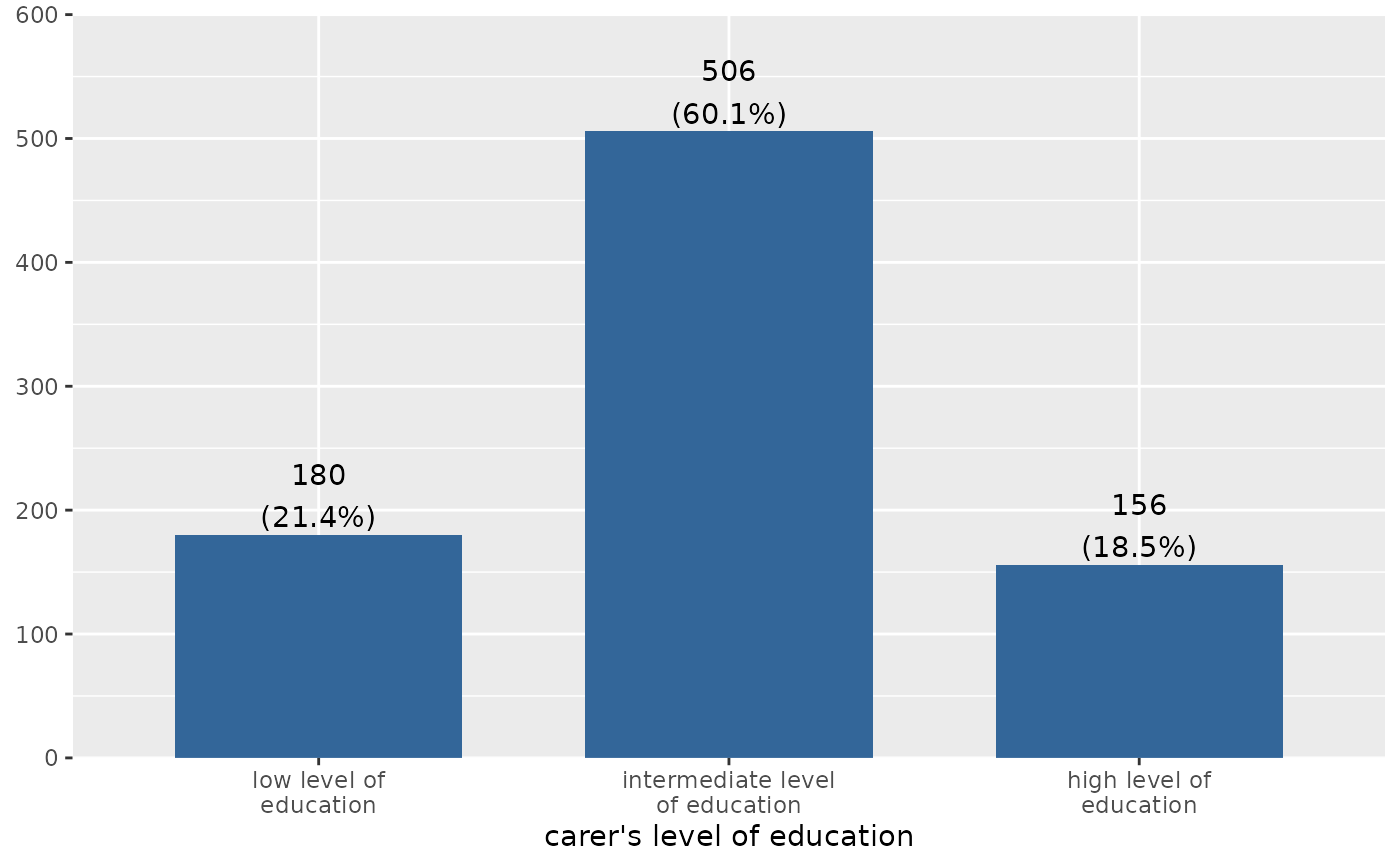#> if (require("dplyr") && require("gridExtra")) { # grouped data frame, all panels in one plot efc %>% group_by(e42dep) %>% plot_frq(c161sex) %>% plot_grid() } #> Loading required package: gridExtra #> #> Attaching package: ‘gridExtra’ #> The following object is masked from ‘package:dplyr’: #> #> combine #> Warning: Not enough tags labels in list. Using letters instead.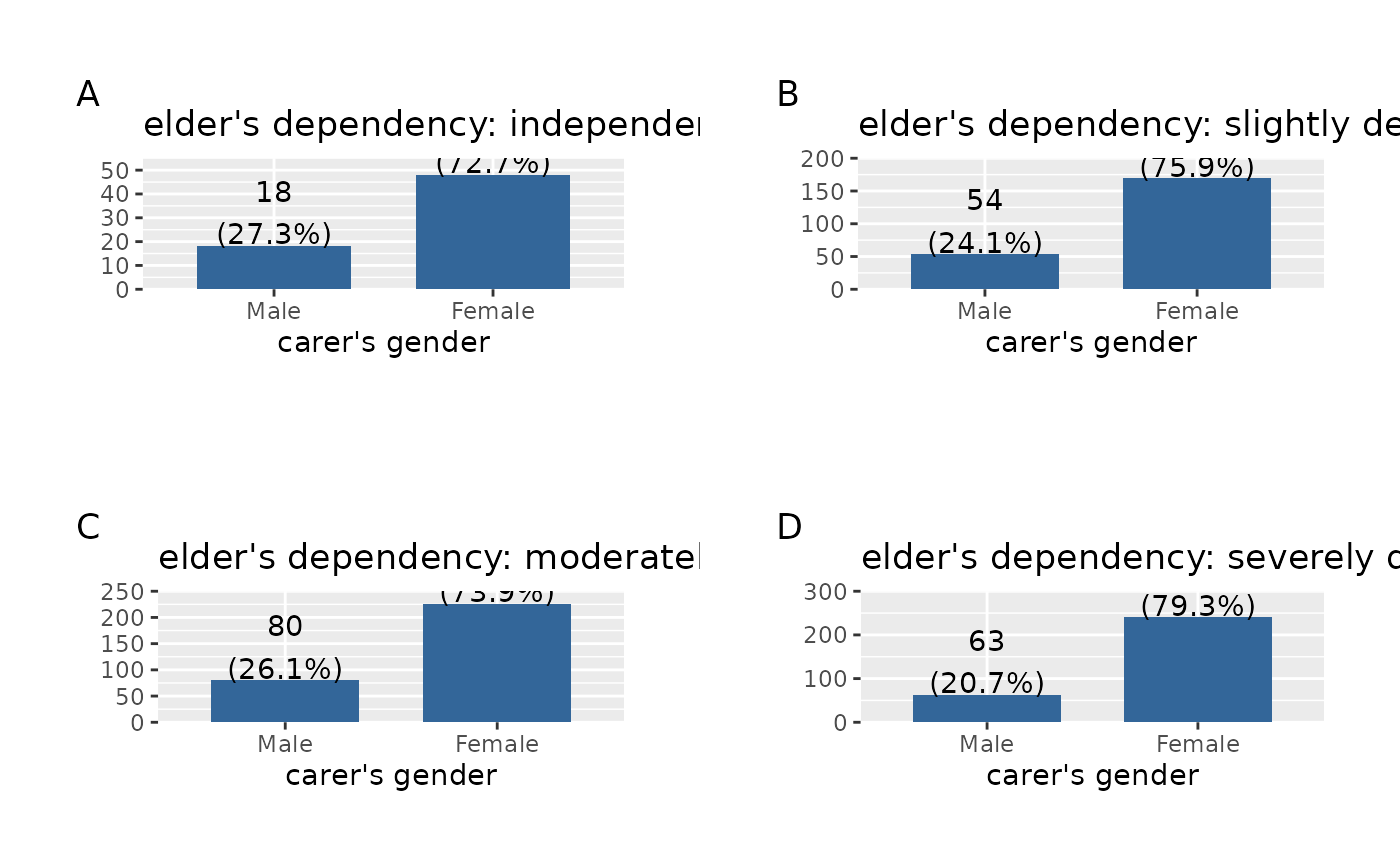# \donttest{ library(sjmisc) # grouped variable ageGrp <- group_var(efc$e17age)
ageGrpLab <- group_labels(efc$e17age) plot_frq(ageGrp, title = get_label(efc$e17age), axis.labels = ageGrpLab)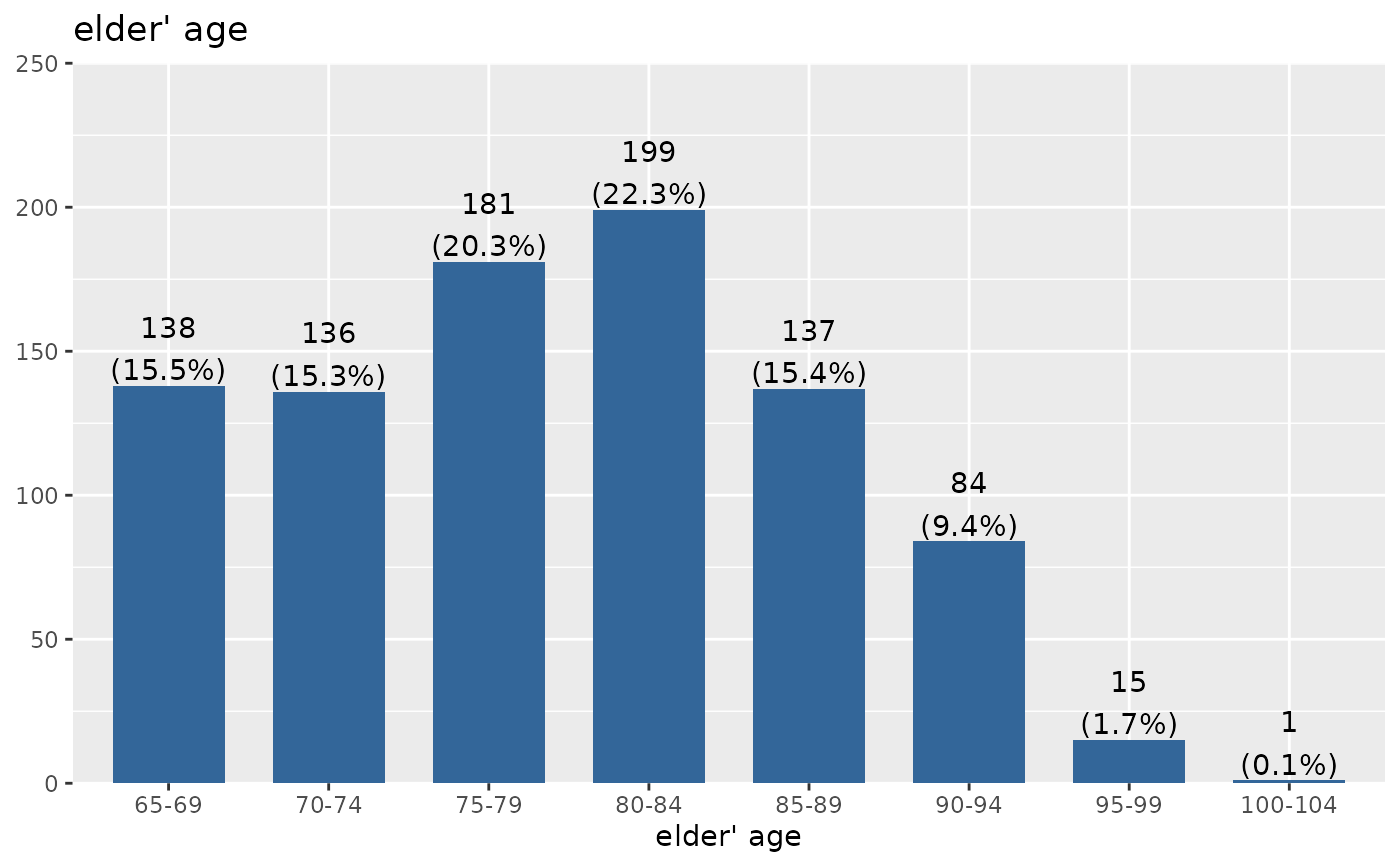# plotting confidence intervals. expand grid and v/hjust for text labels
plot_frq(
efc$e15relat, type = "dot", show.ci = TRUE, sort.frq = "desc", coord.flip = TRUE, expand.grid = TRUE, vjust = "bottom", hjust = "left" )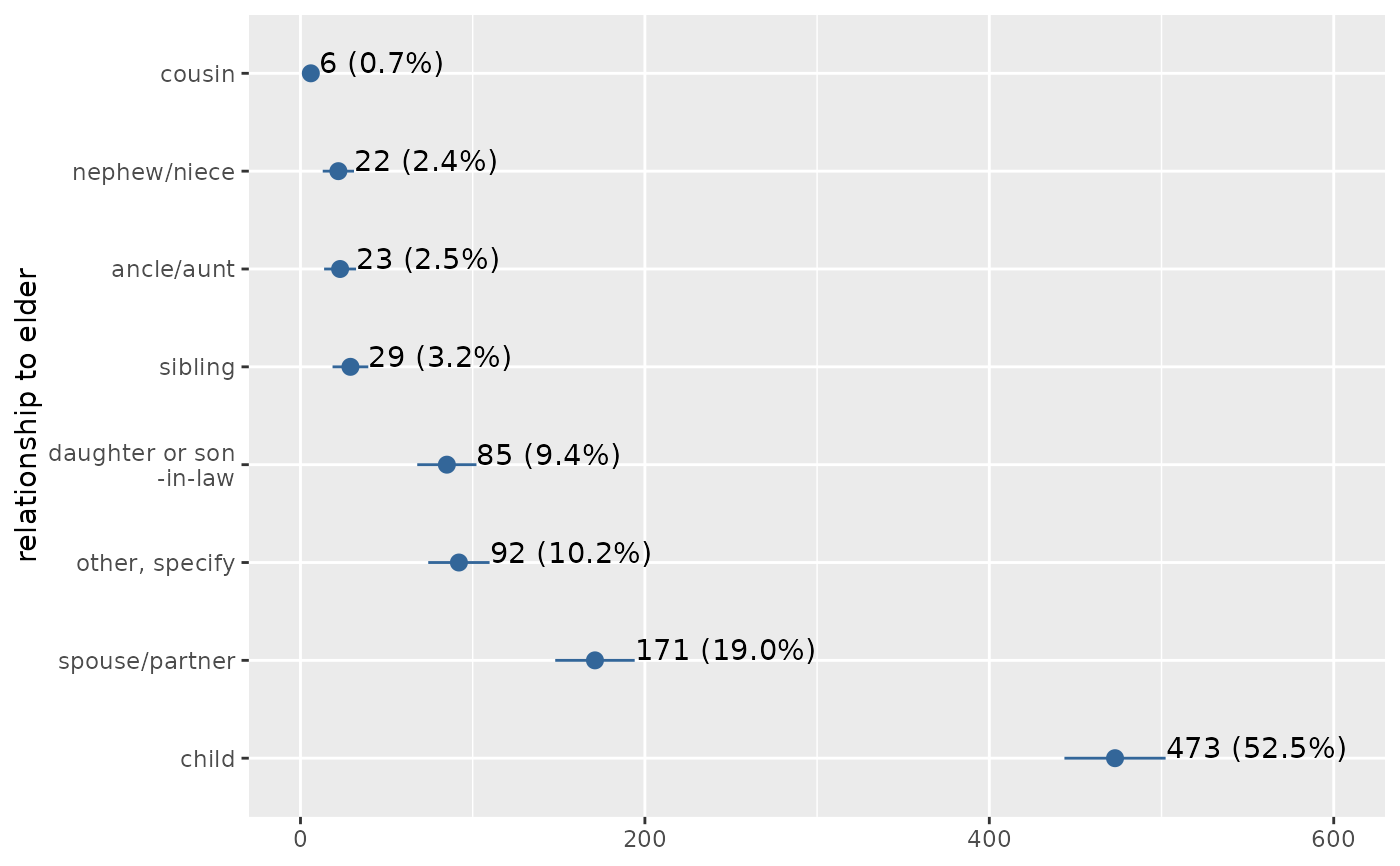# histogram with overlayed normal curve plot_frq(efc$c160age, type = "h", show.mean = TRUE, show.mean.val = TRUE,
normal.curve = TRUE, show.sd = TRUE, normal.curve.color = "blue",
normal.curve.size = 3, ylim = c(0,50))
#> Warning: Removed 4 rows containing missing values (geom_bar()).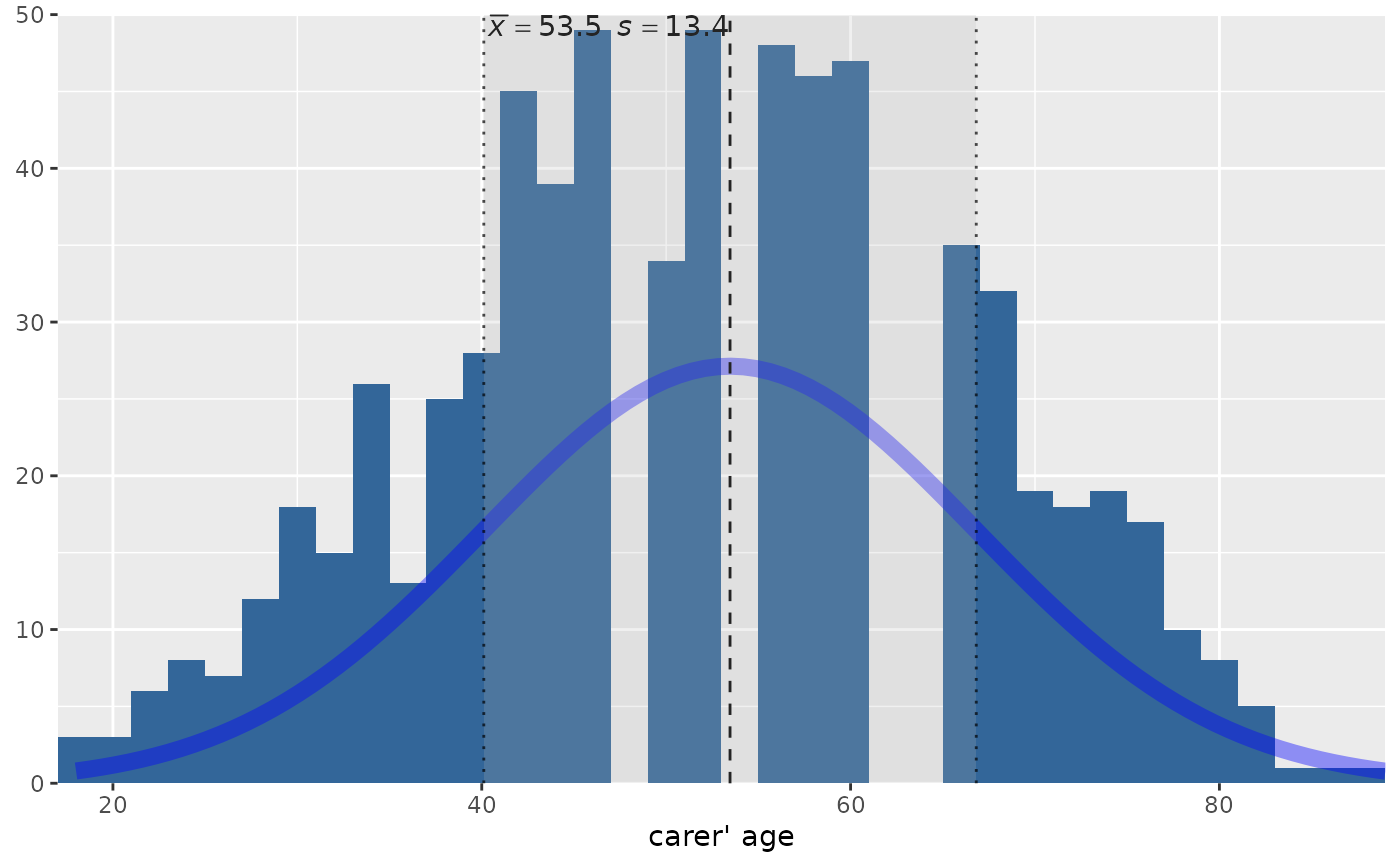# }